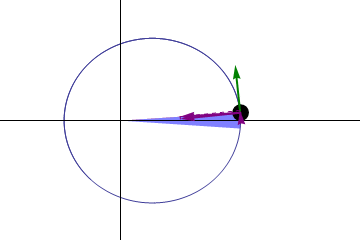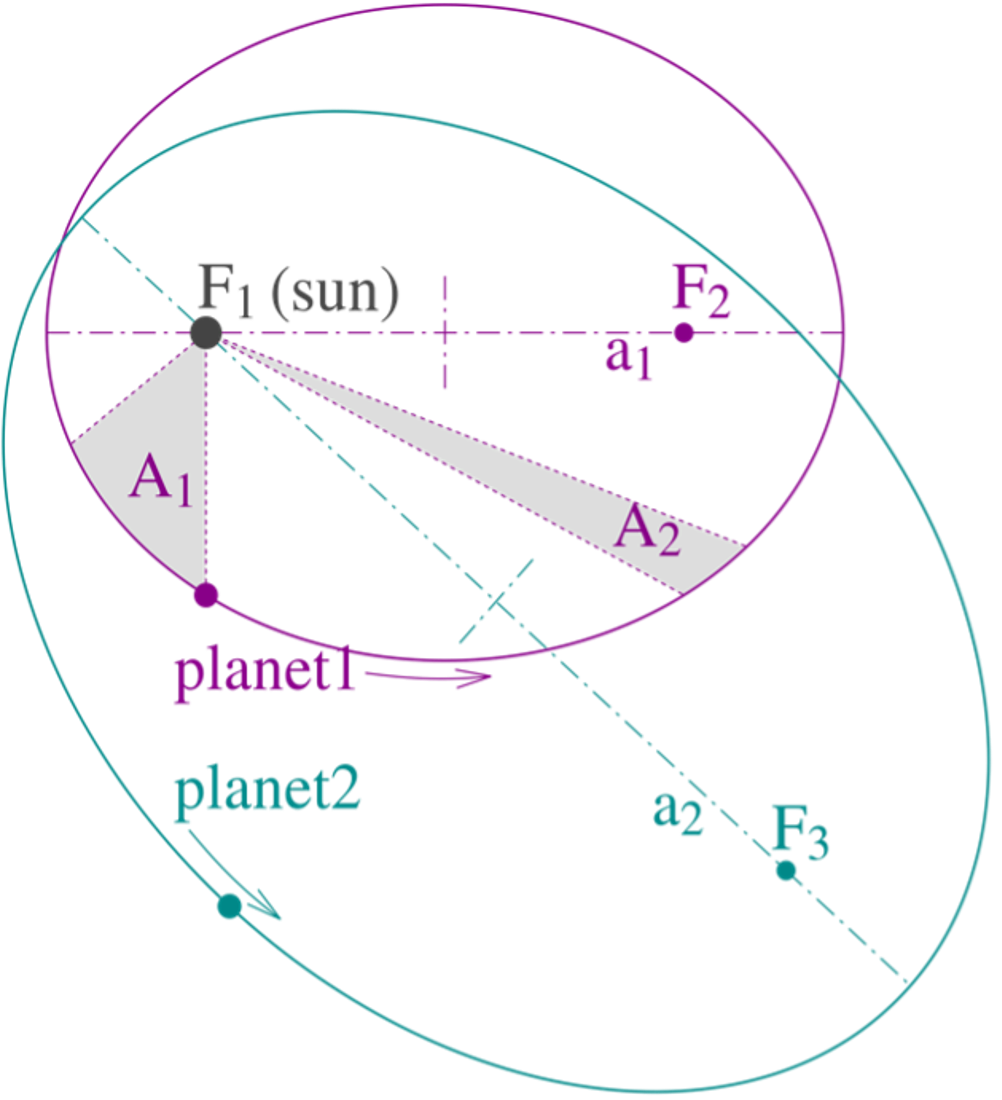## Planetary Orbits

Sun Shines in High-Energy X-rays. Image Credits: NASA NuSTAR

While methods to predict solar eclipses have been known for a long time, the science of how solar eclipses occur is based on Newton’s laws of gravitation and Kepler’s laws of planetary orbits:

Kepler’s three laws describe how planetary bodies orbit the Sun. Here, we introduce these laws.

## Planets move in elliptical orbits with the Sun as a focusThe Earth orbits around the Sun and the Moon orbits the Earth. Both bodies take elliptical trajectories. Image Credits: NOAA, NASA.

The shape that planets orbit their respective star is not a circle, it is an ellipse (similar to an oval). When the planet is at its closest to the star, we call this point the perihelion. When it is at its furthest away, this point is called the aphelion. This law is also applied to natural satellites such as the Moon.

## A line segment joining a planet and the Sun sweeps out equal areas during equal intervals of time.Kepler’s second law for a planetary orbit. Credits: Gonfer (created with Mathematica)

The orbital radius and angular velocity of the planet in the elliptical orbit will vary. This is shown in the animation: the planet travels faster when closer to the Sun, then slower when farther from the Sun. Kepler’s second law states that the blue sector has a constant area.

## A planet’s orbital period is proportional to the size of its orbit (its semi-major axis).

That is,

T2 ∝ a3Illustration of Kepler’s three laws with two planetary orbits. Credits: Han-Kwang Nienhuys.

## Newton’s law of gravitation

Newton’s law of gravitation states that: –

“Every point mass attracts every other point mass by a force acting along the line intersecting the two points. The force is proportional to the product of the two masses, and inversely proportional to the square of the distance between them.”

This is mathematically expressed as: –

F = (Gm1m2) / r2

where

• F is the force between the masses;
• G is the Newtonian constant of gravitation (6.674×10−11 m3⋅kg−1⋅s−2);
• m1 is the first mass;
• m2 is the second mass;
• r is the distance between the centres of the masses.

Newton, in 1687, showed that relationships like Kepler’s would apply in the Solar System as a consequence of his own laws of motion and law of universal gravitation.

Thus, with Newton’s Law of Gravitation explaining the reason for the attraction between 2 planets (which General Relativity extends to describe the nature of spacetime and the phenomenon of Gravitational lensing) and Kepler’s laws of motion describing the motion of these bodies, we can adequately describe how the moon revolves around the Earth.

What makes solar eclipses possible is the occurrence of a cosmic coincidence. The Moon is approximately 400 times smaller than the Sun but the Moon is 389 times closer to the Earth on average which makes solar (particularly total) eclipses possible!

The orbital mechanics of the Earth, Moon, and Sun system can be approximately described by these few laws but if all of these were the only true factors, there should be every 29 days (described by the phases of the moon). This isn’t true and that’s due to the interplay of several factors, which are summarized below.

Summary Point
The Moon revolves around the Earth in an ellipse and the Earth revolves around the Sun in an ellipse and this causes the Moon to revolve a bit more to compensate for the Earth’s revolution.
The Moon’s orbit around the Earth is titled at an angle of 5°. Therefore, we must calculate the amount of time it takes for the Moon to go down and up the Earth’s orbit which is about 27.21221 days. This would give us about 2 eclipses a year.
The Moon’s orbit drifts by 40.7 degrees every year (it’s orbit orbits) so it rotates once every 8.85 years.
The same applies to the Earth’s elliptical orbit which rotates around the Sun 25000 years.
The Earth’s surface complicates this since it’s an obolid (and not a sphere) which is covered with water whose tidal drag causes a difference in this orbit.
The Moon’s speed constantly changes as it travels in this orbit (as a consequence of what is essentially – the three-body problem) which is why all we can really do is take averages of the velocity, trajectory and time amongst other factors.

In summary, we cannot know all the eclipses that will happen with certainty over time, but we can predict it to a nearer estimate. Therefore, the laws of Orbital Mechanics easily explain the occurrence of solar eclipses, but it gets very complicated due to the above-mentioned factors and leads to a beautiful dance between the Earth, Moon, and Sun!

“Kepler’s Laws”hyperphysics.phy-astr.gsu.edu. (Accessed: 30th June 2023).

https://en.wikipedia.org/wiki/User:Gonfer, Animation of Kepler’s second law for a planetary orbit (2010). Available at: https://upload.wikimedia.org/wikipedia/commons/6/69/Kepler-second-law.gif (Accessed: 30th June 2023)

Hankwang (2007), Diagram illustrating Kepler’s laws. (Accessed: 30th June, 2023)

 Proposition 75, Theorem 35: p. 956 – I.Bernard Cohen and Anne Whitman, translators: Isaac NewtonThe PrincipiaMathematical Principles of Natural Philosophy. Preceded by A Guide to Newton’s Principia, by I. Bernard Cohen. University of California Press 1999 ISBN 0-520-08816-6 ISBN 0-520-08817-4

Sagredo (2008), Geometry of a Total Solar Eclipse,  (Accessed: 30th June 2023)

Harrington, Philip S. (1997). Eclipse! The What, Where, When, Why and How Guide to Watching Solar and Lunar Eclipses. New York: John Wiley and Sons. ISBN 0-471-12795-7.

Steel, Duncan (1999). Eclipse: The celestial phenomenon which has changed the course of history. London: Headline. ISBN 0-7472-7385-5.

Last Updated: